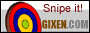back## Microscope Basics

• Another (pr68.com) attempt at basics
• Aberrations - spherical, chromatic
• Aperture - effective/working, equivalent, pupil ratio, vs numerical (N.A.)
• Camera + Microscope Combinations
• Canon EF DSLR sensor-to-flange depth
• Condensers
• Contrast and non-image-forming light:  Kohler
• Depth-of-Focus scaling
• Diopter vs focal length
• Kohler illumination
• Lens formulae
• Magnification:  Classic compound microscope
• Nikon's extensive Interactive Tutorials
• Objectives:  finite vs infinity-corrected
• Zerene focus stacking
• 1:4 to 8:1 magnification FAQ

### Finite vs infinity-corrected objectives

To prime your thinking about lenses and ray diagrams, review this explanation by Bill Otto.Finite-conjugate microscope system vs. infinite-conjugate microscope system with tube lens.
from:  Systematic design of microscope objectives
The vertical dotted line where rays cross at right end of tube length is called the objective's rear conjugate.
To the extent that it is, a finite objective's correction is designed for that tube length,
keeping in mind than many objectives also depend on both slide cover glass and eyepiece for correction.

#### More explanation

The upper diagram is supposed to represent a traditional (RMS == Royal Microscope Society) microscope.
"tube length" is approximately the objective's focal length for its specified magnification,
if that objective was a simple convex lens.
The lower diagram, which is nominally about more modern microscopes,
is arguably also more accurate for some "finite" microscopes,
if `Objective` + `Infinity space` + `Tube lens` are considered together as a compensated "finite" objective.
While infinity objectives can be used with a tube lens of any focal length,
specified magnification depends on that focal length.

### Contrast and non-image-forming light.

While lenses can and do allow images to be focused on your retina,
they do not prevent other photons from also stimulating sensors.
When viewing a three-dimensional scene, perhaps focusing on a near object,
photons scattered from more distant objects may also land on the same photosensors.
Some non-image-forming light can be blocked by an iris diaphragm, as in this diagram:Ignore that Microlens Array. Relay part is afocal photography, where Field Lens is the eyepiece or ocular.

### Kohler illumination:  lamp, field diaphragm and condenser alignment

Any number of websites will waste space pointing out how important it is,
then provide miserable, incomplete and wrong instructions.
Fortunately, Wikipedia does all right;   here are my Kohler experiences.

### Lens formulae

simple
• `1/f = 1/do + 1/di`           {1} `di` becomes `f` for infinite `do`
• `m = di/do`                         {2} zero magnification for lens focused @ infinity
magnification change by focus distance
• `f = (d2 - d1)/(m2 - m1)`; {3} alternatively:
• `d2 = d1 + f*(m2 - m1)`
• `m2 = m1 + (d2 - d1)/f`
magnification for classic (RMS) compound microscope
• `m = (L/fo)*(D/fe)`,
...where:
• `m` = magnification
• `L` = tube length (160mm)
• `D` = normal vision relaxed distance (250mm)
• `f` = focal length
• `fo` = objective focal length
• `fe` = eyepiece focal length
• `di` = lens to image distance
• `do` = lens to object distance
For 160mm tube length, a 10x objective has 16mm focal length
and a 10x eyepiece has 25mm focal length.
For infinity scopes, substitute "tube lens focal length" for "tube length".
Olympus infinity objectives expect 180mm tube lens focal length;
Nikon finite CF BD and M Plan objectives expect 210mm tube length.

### Aberrations

• Spherical lens surfaces are relatively easy to produce, but not ideal.
• Combining different dispersions and refractive indices can reduce lens aberrations.
Compensate vs correct
• correction == positive correction in a downstream optic for upstream optic deficiency.
• compensation == inverse correction in a downstream optic to cancel upstream optic over correction.
Chromatic Aberrations (CA)
• Correcting optics display blue interior CA and yellow exterior CA;  reversed in compensating optics.

### Aperture

effective (working aperture)
• Coupled lenses, stopped in the front, with the rear lens focused at infinity:  `m * lens aperture`
• Single lens, focused by extension:  `(m+1) * lens aperture`
• Teleconverter factor x inserted between camera and all other optics:  `x * lens aperture`
• Microscope objectives used as designed:  `m / (2 * N.A.)`
equivalent e.g. "How does a 4X N.A. 0.1 objective compare to an f/whatever macro lens?"
• `f=1/(2*N.A.)`  is not a bad approximation.
• A better approximation would be `f=1/(2*N.A.) * M/(M+1)`, where `M` is rated magnification.

### aperture vs N.A. :  `N.A. = 1/(2 * f/#)`

 f/# 1.2 1.4 1.8 2 2.8 4 5.6 8 11 16 N.A. 0.4167 0.357 0.417 0.25 0.1786 0.125 0.0893 0.0625 0.045 0.03125

### Canon EF sensor-to-flange depth:  44mm

Add to extension tubes when testing lens focal lengths

### Condensers:  achro, aplanatic, Abbe;  finite vs infinity

Tweaking Abbe condensers
Abbe flaws begin to bother above N.A. 0.6-7; elevate an Abbe to optimize filling the objective's back lens.

### Depth-of-Focus scaling

`DoF2 = DoF1 * (f/#2/f/#1) * (m1/m2)**2`

### Zerene macro step size tables:  magnification vs frame width, magnification vs aperture

• magnification `m = sensor width / frame width`
• DoF (mm) = `0.0022*(((m+1)*f/#)/m)**2`
• DoF (mm) = `0.00055/(N.A.**2)`

### 1:4 to 8:1 magnification FAQ